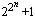# formulas for the primes

The phrase "f(n) is a formula for primes" (where the domain of f is the positive integers) is used in several ways, though most authors mean the first of the following.

I. A function f(n) which takes on all prime values (and only prime values)

Surprisingly, these are relatively easy to construct, though such formulas are usually constructed by first knowing the primes or by counting them. So these formulas are of no value in discovering new primes. See the page "Is there a formula for the nth Prime?" (linked below) and the entry on Bertrand's Postulate for examples.

II. A function f(n) which takes on only prime values

Fermat thought he had an example of this when he declared (falsely) that all the numbers of the formwere primes (these are the Fermat numbers). An obvious (but trivial) example of this is f(n)=2. Mills' Theorem provides a more interesting example.

It is easy to show that no non-constant polynomial (with integer coefficients) can assume only prime values. However, it is possible to construct a polynomial in several integral variables that takes on only primes values (and all of the prime values) when it is positive, but also takes on negative composite values. See Matijasevic's polynomial.

III. A function f(n) which takes on infinitely many prime values (but not only prime values)

It follows from Euclid's theorem that f(n)=n will do. Dirichlet's Theorem also yields such formulae. But even such simple sounding conjectures as "n2+1 is prime infinitely often" seem beyond our reach at present. But there may be some hope, in 1997 Friedlander and Iwaniec showed that there are infinitely many primes among the numbers of the form a2 + b4.

Related pages (outside of this work)

References:

Ribenboim95 (179-212)
P. Ribenboim, The new book of prime number records, 3rd edition, Springer-Verlag, New York, NY, 1995.  pp. xxiv+541, ISBN 0-387-94457-5. MR 96k:11112 [An excellent resource for those with some college mathematics. Basically a Guinness Book of World Records for primes with much of the relevant mathematics. The extensive bibliography is seventy-five pages.]
Wilf82
H. Wilf, "What is an answer?," Amer. Math. Monthly, 89 (1982) 289--292.  MR 83c:05010 [Is there a formula for the primes? Wilf draws a distinction between "formula" and "good formula."]